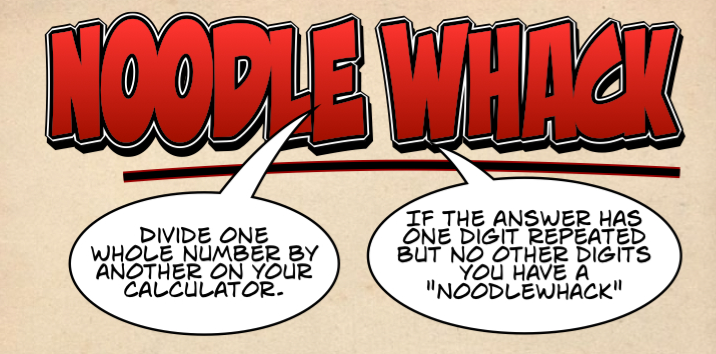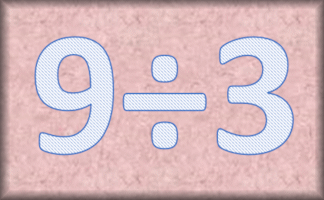#Divide one whole number by another on your calculator. If the answer has one digit repeated but no other digits you have a "Noodlewhack".

## Examples:

 10÷ 3 = 3.3333333 111 ÷5 = 22.2 10÷ 9 = 1.1111111 198 ÷45 = 4.4

## How many Noodlewhacks can you find?

Topics: Starter | Arithmetic | Calculator | Decimals

• Jonathan Sun, South Yarra.Vic.Australia
•
• 10 divide by 9 = 1.11111111111
10 divide by 8 = 1.25
10 divide by 7 = 1.42857142857
10 divide by 6 = 1.66666666666
10 divide by 4 = 2.5
10 divide by 3 = 3.33333333333
I learn how to work out noodle whacks since I was 6.
• Nate, Mount Erin College
•
• 20/18=1.111
30/27=1.111
40/36=1.111
Repeat :).

How did you use this starter? Can you suggest how teachers could present or develop this resource? Do you have any comments? It is always useful to receive feedback and helps make this free resource even more useful for Maths teachers anywhere in the world.

Previous Day | This starter is for 3 January | Next Day

The name of this starter is based on Googlewhack.
Type two words into the Google search engine. If Google comes back with only one hit, you have a Googlewhack.

Your access to the majority of the Transum resources continues to be free but you can help support the continued growth of the website by doing your Amazon shopping using the links on this page. Below is an Amazon link. As an Amazon Associate I earn a small amount from qualifying purchases which helps pay for the upkeep of this website.

Educational Technology on AmazonTeacher, do your students have access to computers such as tablets, iPads or Laptops?  This page was really designed for projection on a whiteboard but if you really want the students to have access to it here is a concise URL for a version of this page without the comments: Transum.org/go/?Start=January3 However it would be better to assign one of the student interactive activities below.Here is the URL which will take them to a related student activity.

Transum.org/go/?to=DividesExactlyFor All: